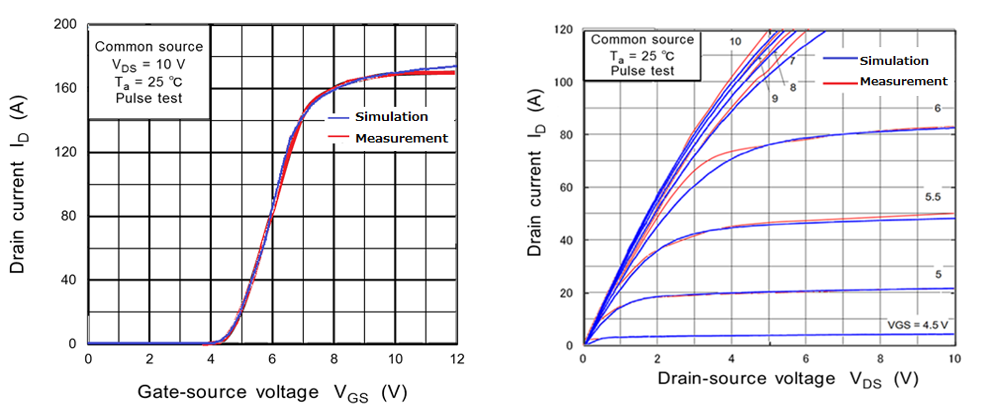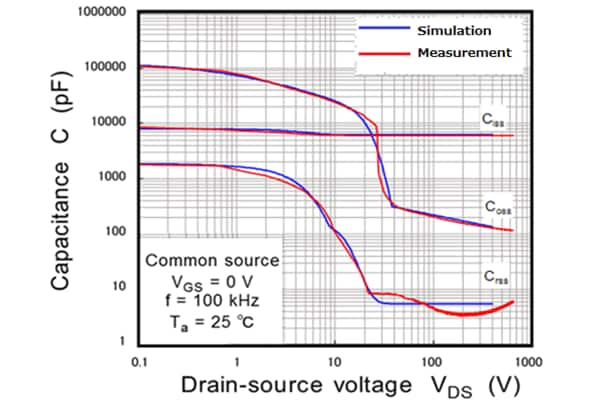# High accurate SPICE model for medium to high voltage MOSFET (400V-900V)

## Structure and features

As shown in Fig. 1, Toshiba’s DTMOS series applies Superjunction (SJ) structure in which P and N layers are alternately arranged in the drift layer. The high accurate SPICE model (G2 model) for SJ structure MOSFET (SJ-MOSFET) developed by Toshiba can express the saturation characteristics in the high current region of Drain current vs Drain-source Voltage (ID-VDS) charactaristics. And the abrupt capacitance change depending on the MOSFET Drain voltage shown in Fig. 2 is also reproduced by using the arbitrary function.

## MOSFET fitting accuracy

Fig. 3 shows the reproduction level (called "fitting accuracy") by comparing the actual measurement of ID-VGS characteristics and ID-VDS characteristics of our SJ-MOSFET product TK040N65Z with the simulation using our SPICE model. In the High voltage breakdown MOSFET such as SJ-MOSFET,  Drain Current (ID) saturate due to the resistance effected by the deep and long drift layer. In our SPICE model, this saturation characteristic is expressed by a non-linear resister (RX) which applied to the drain side so the simulation curve can show actual measurement with accuracy even in the high current region.Fig. 3: Comparison of the actual measurement and simulation of the G2 model (ID-VGS and ID-VDS)

Fig. 4 shows a comparison between actual measurement and simulation of the VDS dependencies on the Input capacitance (Ciss), the Output capacitance (Coss), and the Reverse transfer capacitance (Crss). The capacitance characteristics of SJ-MOSFET change rapidly with the depletion layer extending from the PN-junction due to the SJ structure. On the other hand, Toshiba's SPICE model can show the non-linearity of the measurement values with high accuracy by additional arbitrary functions of non-linear capacitance combination of Gate-souece (Cgs), Gate-drain (Cgd) and Drain-source (Cds).Fig. 4: Comparison of the actual measurement and simulation of the G2 model (Capacitance characteristics)

## Transient characteristics (inductance load switching operation) fitting accuracy

Fig. 5 shows a inductive load switching circuit diagram using SJ-MOSFET product TK040N65Z. And Fig. 6 shows the comparison between the actual waveform and the simulation using the G2 model for SJ-MOSFET as described above. By using our G2 model which can express the non-linearity of the capacitance characteristic with high accuracy, we can see very accurate waveforms that the rising of VDS and oscillation of VDS and ID, associated with oscillation of dVDS/dt or VGS. Our G2 model for SJ-MOSFET is a SPICE model that can sufficiently verify dynamic properties such as switching operation.